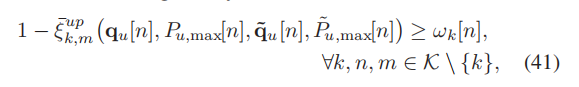# For help!How to express the following formula in CVX?

In the following formula, k represents any integer in 1-4, m represents any integer other than k in 1-4, and n represents any integer in 1-240. In my opinion, the left is a three-dimensional matrix and the right is a two-dimensional matrix. How can I express that the left and right sides are equal?This might help